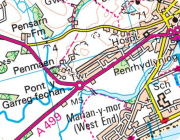# Pocket 'walking' GPS

## Introduction

In the UK, all the walking maps are specially aligned with OS Grid References, so given one number you can look up exactly where you are.

This is extremely useful, and in fact most GPS's have the functionality built in. However often it requires a lot of messing around with buttons to get to the right menu. What if you wanted a simple, small device that did just one thing: displaying OS coordinates of your location in large, easily readable text?

## Wiring Up

Simply connect both the GPS and the Nokia LCD as described in their pages. That's it!

## Software

After a quick google, I found this great webpage which contains the JavaScript code needed to convert between normal Latitude and Longitude and OS map coordinates. This actually makes the whole task really easy - the code is below:

``````/* - - - - - - - - - - - - - - - - - - - - - - - - - - - - - - - - - - - - - - - - - - - - - - -  */
/*  Ordnance Survey Grid Reference functions  (c) Chris Veness 2005-2014                          */
/*   - www.movable-type.co.uk/scripts/gridref.js                                                  */
/*   - www.movable-type.co.uk/scripts/latlon-gridref.html                                         */
/* - - - - - - - - - - - - - - - - - - - - - - - - - - - - - - - - - - - - - - - - - - - - - - -  */

function OsGridRef(easting, northing) {
this.easting = 0|easting;
this.northing = 0|northing;
}

OsGridRef.latLongToOsGrid = function(point) {
var lat = point.lat.toRad();
var lon = point.lon.toRad();

var a = 6377563.396, b = 6356256.909;          // Airy 1830 major & minor semi-axes
var F0 = 0.9996012717;                         // NatGrid scale factor on central meridian
var lat0 = (49).toRad(), lon0 = (-2).toRad();  // NatGrid true origin is 49�N,2�W
var N0 = -100000, E0 = 400000;                 // northing & easting of true origin, metres
var e2 = 1 - (b*b)/(a*a);                      // eccentricity squared
var n = (a-b)/(a+b), n2 = n*n, n3 = n*n*n;

var cosLat = Math.cos(lat), sinLat = Math.sin(lat);
var nu = a*F0/Math.sqrt(1-e2*sinLat*sinLat);              // transverse radius of curvature
var rho = a*F0*(1-e2)/Math.pow(1-e2*sinLat*sinLat, 1.5);  // meridional radius of curvature
var eta2 = nu/rho-1;

var Ma = (1 + n + (5/4)*n2 + (5/4)*n3) * (lat-lat0);
var Mb = (3*n + 3*n*n + (21/8)*n3) * Math.sin(lat-lat0) * Math.cos(lat+lat0);
var Mc = ((15/8)*n2 + (15/8)*n3) * Math.sin(2*(lat-lat0)) * Math.cos(2*(lat+lat0));
var Md = (35/24)*n3 * Math.sin(3*(lat-lat0)) * Math.cos(3*(lat+lat0));
var M = b * F0 * (Ma - Mb + Mc - Md);              // meridional arc

var cos3lat = cosLat*cosLat*cosLat;
var cos5lat = cos3lat*cosLat*cosLat;
var tan2lat = Math.tan(lat)*Math.tan(lat);
var tan4lat = tan2lat*tan2lat;

var I = M + N0;
var II = (nu/2)*sinLat*cosLat;
var III = (nu/24)*sinLat*cos3lat*(5-tan2lat+9*eta2);
var IIIA = (nu/720)*sinLat*cos5lat*(61-58*tan2lat+tan4lat);
var IV = nu*cosLat;
var V = (nu/6)*cos3lat*(nu/rho-tan2lat);
var VI = (nu/120) * cos5lat * (5 - 18*tan2lat + tan4lat + 14*eta2 - 58*tan2lat*eta2);

var dLon = lon-lon0;
var dLon2 = dLon*dLon, dLon3 = dLon2*dLon, dLon4 = dLon3*dLon, dLon5 = dLon4*dLon, dLon6 = dLon5*dLon;

var N = I + II*dLon2 + III*dLon4 + IIIA*dLon6;
var E = E0 + IV*dLon + V*dLon3 + VI*dLon5;

return new OsGridRef(E, N);
};

// ----------------------------------- One wrapper function needed to make it work...
Number.prototype.toRad = function() {
return this*Math.PI/180;
};

SPI3.setup({ baud: 1000000, sck:B3, mosi:B5 });
var g;
function onInit() {
// We set up the LCD here because it needs to initialise at power on
g = require("PCD8544").connect(SPI3,B6,B7,B8, function () {
// called when initialised...
g.clear();
g.flip();
});
}

Serial4.setup(9600,{tx:C10,rx:C11});
var gps = require("GPS").connect(Serial4, function(data) {
var os = OsGridRef.latLongToOsGrid(data);
g.clear();
g.setFontBitmap();
g.drawString("Northing", 10,0);
g.drawString("Easting", 10,24);
g.setFontVector(14);
g.drawString(os.northing, 0,5);
g.drawString(os.easting, 0,29);
g.flip();
});

onInit();
``````

This page is auto-generated from GitHub. If you see any mistakes or have suggestions, please let us know.